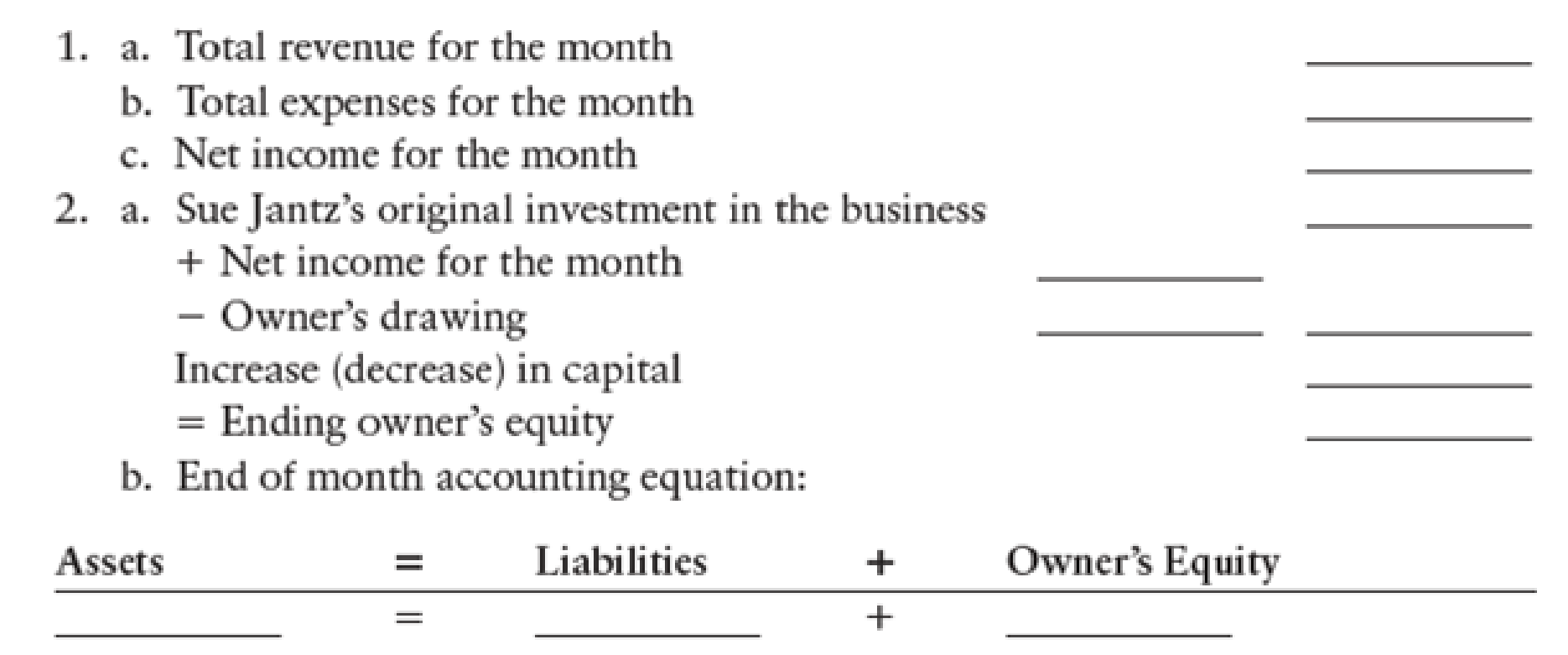Chapter 3, Problem 14SPB

Chapter
Section
Textbook Problem

NET INCOME AND CHANGE IN OWNER’S EQUITY Refer to the trial balance of Jantz Plumbing Service in Problem 3-13B to determine the following information. Use the format provided below.1. a

To determine

Determine the total revenue for the month of August.

Explanation

Revenue is known as “Top line” because it appears very top on the income statement of the company. It is used to imply profits or net income (bottom line) of the company by subtracting the total expenses from total revenues.

Determine the total revenue for the month of August:

Total revenue for the month of August = (Service revenue +</

1.b

To determine

Determine the total expenses for the month of August.

1.c

To determine

Determine the net income for the month of August.

2.a

To determine

Determine the amount of ending owner’s equity.

2.b

To determine

Show the accounting equation for the end of month.

Still sussing out bartleby?

Check out a sample textbook solution.

See a sample solution

The Solution to Your Study Problems

Bartleby provides explanations to thousands of textbook problems written by our experts, many with advanced degrees!

Get Started

Find more solutions based on key concepts